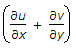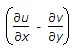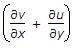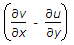# Civil Engineering - UPSC Civil Service Exam Questions

11.

Which one of the following statements is correct ?
Lug angles:

 A. are necessarily unequal angles B. are always equal angles C. increase the shear resistance of joint D. reduce the length of joint

Explanation:

No answer description available for this question. Let us discuss.

12.

Consider the following statements :
A : The collapse load found in the plastic analysis of a continuous beam is affected by sinking of supports.
B : Large elastic stresses are caused at the supports due to sinking of supports.
Of these statements :

 A. Both A and B are true B. A is true but B is false C. Both A and B are false D. A is false but B is true

Explanation:

No answer description available for this question. Let us discuss.

13.

When a particular discharge is flowing in a horizontal pipe, a mercury-water U-tube manometer connccted to the entrance and throat of a venturimeter fitted in the pipe recorded a deflection of 25 cm. If the same discharge flowed through the same pipe kept at an inclination of 45° to the horizontal, then the corresponding deflection recorded by the U-tube manometer will be

 A. 25 2 cm B. 25/2 cm C. 25/2 cm D. 25 cm

Explanation:

No answer description available for this question. Let us discuss.

14.

Velocity in the z direction is given by

 A.B.C.D.Explanation:

No answer description available for this question. Let us discuss.

15.

A grinding wheel rotates 3000 rpm. When power supply is cut off, the wheel stops completely in 10 seconds. What is the number of revolutions made by the wheel before coming to rest ?

 A. 3000 B. 1000 C. 500 D. 250